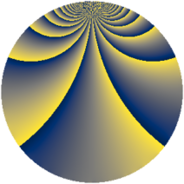# Properties

 Label 2001.2.mLevel $2001$ Weight $2$ Character orbit 2001.m Rep. character $\chi_{2001}(139,\cdot)$ Character field $\Q(\zeta_{7})$ Dimension $648$ Sturm bound $480$

# Related objects

## Defining parameters

 Level: $$N$$ $$=$$ $$2001 = 3 \cdot 23 \cdot 29$$ Weight: $$k$$ $$=$$ $$2$$ Character orbit: $$[\chi]$$ $$=$$ 2001.m (of order $$7$$ and degree $$6$$) Character conductor: $$\operatorname{cond}(\chi)$$ $$=$$ $$29$$ Character field: $$\Q(\zeta_{7})$$ Sturm bound: $$480$$

## Dimensions

The following table gives the dimensions of various subspaces of $$M_{2}(2001, [\chi])$$.

Total New Old
Modular forms 1464 648 816
Cusp forms 1416 648 768
Eisenstein series 48 0 48

## Trace form

 $$648q + 4q^{2} - 104q^{4} + 16q^{5} + 4q^{6} + 8q^{7} + 12q^{8} - 108q^{9} + O(q^{10})$$ $$648q + 4q^{2} - 104q^{4} + 16q^{5} + 4q^{6} + 8q^{7} + 12q^{8} - 108q^{9} + 16q^{10} + 24q^{13} + 16q^{14} - 20q^{15} - 96q^{16} + 48q^{17} + 4q^{18} - 100q^{20} - 20q^{22} + 24q^{24} - 128q^{25} - 60q^{26} - 48q^{28} - 4q^{31} + 28q^{32} + 32q^{33} - 48q^{34} + 32q^{35} - 104q^{36} - 36q^{37} + 16q^{38} + 20q^{40} + 80q^{41} + 20q^{42} + 8q^{43} + 56q^{44} - 40q^{45} - 16q^{47} - 28q^{49} - 80q^{50} - 20q^{51} + 76q^{52} - 56q^{53} + 4q^{54} + 28q^{55} - 92q^{56} + 16q^{57} - 64q^{58} - 24q^{59} - 180q^{60} + 48q^{61} - 116q^{62} - 20q^{63} + 28q^{64} - 72q^{65} - 120q^{66} - 16q^{67} - 132q^{68} + 56q^{70} + 8q^{71} + 12q^{72} - 156q^{73} + 64q^{74} + 16q^{75} + 4q^{76} - 36q^{77} + 108q^{78} + 24q^{79} + 144q^{80} - 108q^{81} + 24q^{82} - 72q^{83} + 48q^{84} + 88q^{85} + 88q^{86} + 16q^{87} + 16q^{88} + 96q^{89} + 16q^{90} + 80q^{91} + 8q^{93} - 48q^{94} + 64q^{95} - 48q^{96} + 8q^{97} - 36q^{98} + O(q^{100})$$

## Decomposition of $$S_{2}^{\mathrm{new}}(2001, [\chi])$$ into newform subspaces

The newforms in this space have not yet been added to the LMFDB.

## Decomposition of $$S_{2}^{\mathrm{old}}(2001, [\chi])$$ into lower level spaces

$$S_{2}^{\mathrm{old}}(2001, [\chi]) \cong$$ $$S_{2}^{\mathrm{new}}(29, [\chi])$$$$^{\oplus 4}$$$$\oplus$$$$S_{2}^{\mathrm{new}}(87, [\chi])$$$$^{\oplus 2}$$$$\oplus$$$$S_{2}^{\mathrm{new}}(667, [\chi])$$$$^{\oplus 2}$$